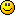Topic: Flight Distance between points

Hi

When using OnlineMapsUtils.DistanceBetweenPoint (http://infinity-code.com/doxygen/online … dd617a6d18)

To get the actual distance between the two point, sqrt(dx*dx+dy*dy) should give the correct value in km ?

Now if i want the distance between two points, which are at different altitude:

OnlineMapsUtils.DistanceBetweenPoint give me the distance between those two point at ground 0, so i was planning to use a simple Pythagore formula to get the "real" distance.

But, if i'm not mistaken, OnlineMapsUtils.DistanceBetweenPoint doesn't calculate a straight line lentgh but an arc (since the earth is not flat and we are using lat/lng )
So Pythagore doesn't seems like a correct way to go ?
How should i proceed to get a correct flight distance between two waypoints at different altitude ?

Re: Flight Distance between points

Hello.

Something like that:

/// <summary>
/// The distance between two geographical coordinates with altitude.
/// </summary>
/// <param name="x1">Longitude 1</param>
/// <param name="y1">Latitude 1</param>
/// <param name="a1">Altutude 1 (km)</param>
/// <param name="x2">Longitude 2</param>
/// <param name="y2">Latitude 2</param>
/// <param name="a2">Altitude 2 (km)</param>
/// <param name="dx">Distance longitude (km)</param>
/// <param name="dy">Distance latitude (km)</param>
public static void DistanceBetweenPoints(double x1, double y1, float a1, double x2, double y2, float a2, out double dx, out double dy)
{
double r = 6371 + (a1 + a2) / 2;
double Deg2Rad = Math.PI / 180;
double scfY = Math.Sin(y1 * Deg2Rad);
double sctY = Math.Sin(y2 * Deg2Rad);
double ccfY = Math.Cos(y1 * Deg2Rad);
double cctY = Math.Cos(y2 * Deg2Rad);
double cX = Math.Cos((x1 - x2) * Deg2Rad);
double sizeX1 = Math.Abs(r * Math.Acos(scfY * scfY + ccfY * ccfY * cX));
double sizeX2 = Math.Abs(r * Math.Acos(sctY * sctY + cctY * cctY * cX));
dx = (sizeX1 + sizeX2) / 2.0;
dy = r * Math.Acos(scfY * sctY + ccfY * cctY);
if (double.IsNaN(dy)) dy = 0;
}
Kind Regards,
Infinity Code Team.

Do not know the best way to thank the developer? Rate the asset in Asset Store!

Re: Flight Distance between points

Thanks a lot